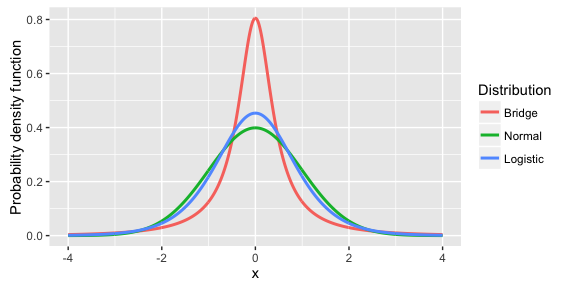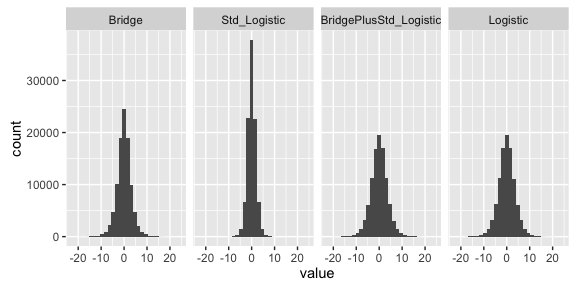library(bridgedist)

Recreate the plot from Wang and Louis (2003) where the Bridge, Normal, and Logistic all have unit variance and mean 0 with ggplot2:

library(reshape2)
library(ggplot2)
xaxis = seq(-4,4,.01)
df = data.frame( xaxis,
Bridge = dbridge(xaxis, scale=1/sqrt(1+3/pi^2)),
Normal = dnorm(xaxis),
Logistic = dlogis(xaxis, scale=sqrt(3/pi^2)))
melt.df <- melt(df, id.vars = "xaxis")
colnames(melt.df) <- c("x", "Distribution", "value")
ggplot(melt.df, aes(x, value, color=Distribution)) +
geom_line(size=1.05) +
ylab("Probability density function")Fig. 1. Probability density functions of the Gaussian, logistic and bridge, for logistic, distributions each with zero mean and unit variance.

The implication is that a random variable from a Bridge distribution plus random variable from a standard logistic distribution is a logistic random variable with a scale greater than one.

phi <- 0.5
df = data.frame(
Bridge = rbridge(1e5, scale=phi),
Std_Logistic = rlogis(1e5),
BridgePlusStd_Logistic = rbridge(1e5, scale=phi) +  rlogis(1e5),
Logistic = rlogis(1e5, scale=1/phi)
)
melt.df <- melt(df)
colnames(melt.df) <- c("Distribution", "value")
ggplot(melt.df, aes(value)) +
facet_grid(.~Distribution) +
geom_histogram()Fig. 2. 10000 random variates in each panel. From left to right: the bridge distribution, the logistic with scale=1, the sum of the previous two, and the logistic with scale=1/phi. Note how similar the third and fourth panel, the application supporting the theory.